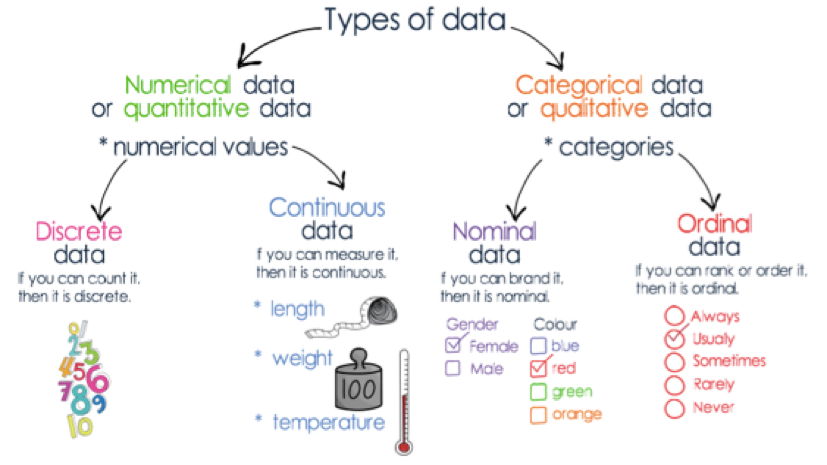# Further Mathematics - Exam Revision## Part 1 - Further Mathematics - Unit 3 - DATA ANALYSIS - Types of data

Authored By Carmen Popescu-Rose 0 Comment(s)

Rule of 👍🏼

If you can calculate the mean of the data, then the data is numerical.

Categorical data written in number form:

* House numbers

* Phone numbers

* Date of birth

* Postcodes

Q1

The variables Country (Australia, China, India) and Surface area (number of square kilometres)

A  are both categorical.

B  are both numerical.

are categorical and numerical respectively.

D  are numerical and categorical respectively.

are categorical nominal and categorical ordinal respectively.

Q2

The variables Month (January, February, March) and Monthly sales (\$)

A  are both categorical.

B  are both numerical.

C  are numerical and categorical respectively.

are categorical and numerical respectively.

are categorical nominal and categorical ordinal respectively.

Q3

The variables level of water usage (L per day) and size of house (m2)

A  are both categorical.

are both numerical.

C  are categorical and numerical respectively.

D  are numerical and categorical respectively.

are neither categorical are both nor numerical.

Q4

The level of water usage of 250 houses was rated in a survey as low, medium or high and

the size of the houses as small, standard or large.

The variables, level of water usage and size of house, as recorded in this survey, are

A  both numerical variables.

B  both categorical variables.

C  neither numerical nor categorical variables.

D  numerical and categorical variables respectively.

categorical and numerical variables respectively.

Q1 C are categorical and numerical respectively.

Q2 D are categorical and numerical respectively.

Q3 B  are both numerical.

Q4 B  both categorical variables.